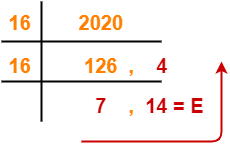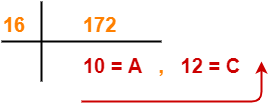## Number System Conversions-

Before you go through this article, make sure that you have gone through the previous article on Basics of Number System.

In number system,

• It is very important to have a good knowledge of how to convert numbers from one base to another base.
• Here, we will learn how to convert any given number from base 10 to base 16.A given number can be converted from base 10 to any other base using division method and multiplication method.

Following two cases are possible-

### Case-01: For Numbers Carrying No Fractional Part-

• Division Method is used to convert such numbers from base 10 to another base.
• The division is performed with the required base.

### Steps To Convert From Base 10 to Base 16-

• Divide the given number (in base 10) with 16 until the result finally left is less than 16.
• Traverse the remainders from bottom to top to get the required number in base 16.

### Case-02: For Numbers Carrying A Fractional Part-

To convert such numbers from base 10 to another base, real part and fractional part are treated separately.

### For Real Part-

The steps involved in converting the real part from base 10 to another base are same as above.

### For Fractional Part-

• Multiplication Method is used to convert fractional part from base 10 to another base.
• The multiplication is performed with the required base.

### Steps To Convert From Base 10 To Base 16-

• Multiply the given fraction (in base 10) with 16.
• Write the real part and fractional part of the result so obtained separately.
• Multiply the fractional part with 16.
• Write the real part and fractional part of the result so obtained separately.
• Repeat this procedure until the fractional part remains 0.
• If fractional part does not terminate to 0, find the result up to as many places as required.

Required Number in Base 16

= Series of real part of multiplication results obtained in the above steps from top to bottom

Also Read- Conversion to Base 10

## Problems-

Convert the following numbers from base 10 to base 16-

1. (2020)10
2. (2020.65625)10
3. (172)10
4. (172.983)10

## Solution-

### 1. (2020)10

(2020)10  (?)16

Using division method, we have-From here, (2020)10 = (7E4)16

### 2. (2020.65625)10

#### (2020.65625)10 → ( ? )8

Here, we treat the real part and fractional part separately-

### For Real Part-

• The real part is (2020)10
• We convert the real part from base 10 to base 16 using division method same as above.

So, (2020)10 = (7E4)16

### For Fractional Part-

• The fractional part is (0.65625)10
• We convert the fractional part from base 10 to base 16 using multiplication method.

Using multiplication method, we have-

 Real part Fractional Part 0.65625 x 16 10 = A 0.5 0.5 x 16 8 0.0

### Step-01:

• Multiply 0.65625 with 16. Result = 10.5.
• Write 10 (= A in hexadecimal) in real part and 0.5 in fractional part.

### Step-02:

• Multiply 0.5 with 16. Result = 8.0.
• Write 8 in real part and 0.0 in fractional part.

Since fractional part becomes 0, so we stop.

• The fractional part terminates to 0 after 2 iterations.
• Traverse the real part column from top to bottom to obtain the required number in base 16.

From here, (0.65625)10 = (0.A8)8

Combining the result of real and fractional parts, we have-

(2020.65625)10 = (7E4.A8)16

### 3. (172)10

#### (172)10 → ( ? )16

Using division method, we have-From here, (172)10 = (AC)16

### 4. (172.983)10

#### (172.983)10 → ( ? )16

Here, we treat the real part and fractional part separately-

### For Real Part-

• The real part is (172)10
• We convert the real part from base 10 to base 16 using division method same as above.

So, (172)10 = (AC)16

### For Fractional Part-

• The fractional part is (0.983)10
• We convert the fractional part from base 10 to base 16 using multiplication method.

Using multiplication method, we have-

 Real part Fractional Part 0.983 x 16 15 = F 0.728 0.728 x 16 11 = B 0.648 0.648 x 16 10 = A 0.368 0.368 x 16 5 0.888

• The fractional part does not terminates to 0 after several iterations.
• So, let us find the value up to 4 decimal places.
• Traverse the real part column from top to bottom to obtain the required number in base 16.

From here, (0.983)10 = (0.FBA5)8

Combining the result of real and fractional parts, we have-

(172.983)10 = (AC.FBA5)16

Also Read- Decimal to Octal Conversion